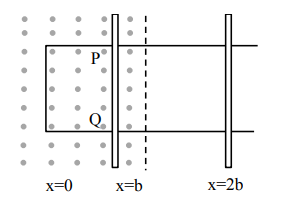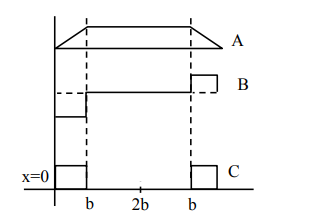# The arm PQ of a rectangular conductor is moving`
Question:

The arm $\mathrm{PQ}$ of a rectangular conductor is moving

from $x=0$ to $x=2 b$ outwards and then inwards

from $x=2 b$ to $x=0$ as shown in the figure. $A$

uniform magnetic field perpendicular to the plane

is acting from $\mathrm{x}=0$ to $\mathrm{x}=\mathrm{b}$. Identify the graph

showing the variation of different quantities with

distance:1. A-Flux, B-Power dissipated, C-EMF

2. A-Power dissipated, B-Flux, C-EMF

3. A-Flux, B-EMF, C-Power dissipated

4. A-EMF, B-Power dissipated, C-Flux

Correct Option: , 3

Solution:

As rod moves in field area increases upto $x=b$ then field is absent and again flux is generated on return journey from $x=b$ to $x=0$. Thus plot $A$ for flux.

$\Rightarrow \mathrm{e}=-\frac{\mathrm{d} \phi}{\mathrm{dt}} \Rightarrow$ curve $\mathrm{B}$ for emf

$\Rightarrow$ Power dissipated $=$ vi $\Rightarrow$ curve $C$ for power dissipated